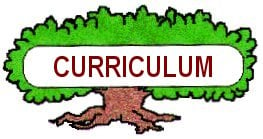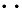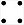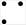Home Page Welcome Page Table of Contents Pre-test Information Tree Basics Section Environment Section Recreation Section Products Section Balance Section Internet Links References Credits Index

MICHIGAN FORESTS FOREVER TEACHERS GUIDE

 STAND VOLUME SHEETDownload the Word document of this Volume Sheet.

# VOLUME SHEET (Record the total number of plots taken)

### Sum of Totals From Each Field Tally Sheet

PULPWOOD VOLUME                                                                    BASAL AREA

 Species #sticks #trees Cords/acre Sq.Ft./acre All Species S S S

SAWTIMBER VOLUME                                                                  BASAL AREA

 Species #logs #trees+ Avg * Factor bdft/acre Sq.Ft./acre All Species S S S

* Compute average # logs per tree  + tree count ONLY for those sawlogs without pulpsticks

 Pulpwood Formula Basal Area Formula #sticks + #trees = cords/acre #tree x 10 = square feet/acre 2 x #plots #plots

 Sawtimber Formula Factors for Average #Logs/Tree #logs x factor = board feet/acre 1 - 375 4 - 313 #plots 2 - 375 5 - 300 3 - 330 6 - 290

#### INSTRUCTIONS

1.  You will need information from each Field Tally Sheet from each crew in order to calculate volumes and basal area for the entire stand of timber.

2.  On the field tally sheet using the dot tally, write a small number in the upper right-hand corner of each box that reflects the number of sticks (or logs) recorded in that box.  Multiply the dot tally by the number in the column heading.

 Species 1 2 3 4 5 6 7 8 #Sticks #Trees r e d   m a p l e 412121543 12

3.  List the species that occurred in the stand.  If there are more species than lines, you can lump the least common species into a miscellaneous category.

4.  Take the total values from each Field Tally Sheet, for each species, and add them together.  Record these sums in the appropriate places on this form.  Values that need to be summed are:  #sticks, #sawlogs, #trees, and the number of plots.  Remember that this must be done for each species.  For example, the total number of pulpsticks recorded for sugar maple from all the Field Tally Sheets is . . .

5.  Calculate the pulpwood volume (cords/acre) for each species using the formula at the bottom of the Field Tally Sheet.

6.  Calculate the basal area (square feet/acre) for each pulpwood species using the formula at the bottom of the Field Tally Sheet.

7.  Calculate the sawtimber volume (board feet/acre) for each species using the formula at the bottom of the Field Tally Sheet.  You will have to first calculate the average number of logs per tree (for each species) by dividing the number of logs by the number of trees.

8.  Calculate the basal area (square feet/acre) for each sawtimber species using the formula at the bottom of the Field Tally Sheet.  Note that the sawtimber basal area is a portion of the pulpwood basal area.  The pulpwood basal area is the total basal area for the stand, unless there is a sawtimber tree with no pulpwood above the sawlog.  This does happen, but it is unusual.  In most cases, there will not be a sawtimber basal area calculation.

9.  Sum the total number of sticks and total number of trees from the entire inventory.  Use these values to calculate the total cords/acre of the stand.  This value should be same as when you add the cords/acre/species in the right-hand column.  This is a good arithmetic check.

10.  Perform the similar calculations for sawtimber volume, again using the right-hand column as an arithmetic check.This website was developed and created by Michigan State University Extension for the teachers of the State of Michigan. .